# registr: a vignette

#### 2020-03-08

The registr package is for registering, or aligning, exponential family functional data.

## What is exponential family registration?

Functional data analysis is a set of tools for understanding patterns and variability in data where the basic unit of observation is a curve measured over some domain such as time or space. An example is an accelerometer study where intensity of physical activity was measured at each minute over 24 hours for 50 subjects. The data will contain 50 curves, where each curve is the 24-hour activity profile for a particular subject.

Classic functional data analysis assumes that each curve is continuous or comes from a Gaussian distribution. However, applications with exponential family functional data – curves that arise from any exponential family distribution, and have a smooth latent mean – are increasingly common. For example, take the accelerometer data just mentioned, but assume researchers are interested in sedentary behavior instead of activity intensity. At each minute over 24 hours they collect a binary measurement that indicates whether a subject was active or inactive (sedentary). Now we have a binary curve for each subject – a trajectory where each time point can take on a value of 0 or 1. We assume the binary curve has a smooth latent mean, which in this case is interpreted as the probability of being active at each minute over 24 hours. This is a example of exponential family functional data.

Often in a functional dataset curves have similar underlying patterns but the main features of each curve, such as the minimum and maximum, have shifts such that the data appear misaligned. This misalignment can obscure patterns shared across curves and produce messy summary statistics. Registration methods reduce variability in functional data and clarify underlying patterns by aligning curves.

At the core of this registration method is generalized functional principal components analysis (GFPCA), a popular technique for extracting patterns shared across curves.

### The registr model and algorithm

The main model for exponential family registration is

$\begin{eqnarray*} E\left[Y_i\left(h_i^{-1}(t_i^*)\right) | c_i, h_i^{-1} \right] &=& \mu_i(t) \\ g\left[\mu_i(t)\right]&=& \alpha(t) + \sum_{k = 1}^K c_{ik}\psi_k(t). \end{eqnarray*}$ For subject $$i$$, inverse warping function $$h_i^{-1}$$ maps unregistered time $$t_i^*$$ to registered time $$t$$ such that $$h_i^{-1}(t_i^*) = t$$. $$Y_i\left(t_i^*\right)$$ and $$Y_i\left(h_i^{-1}(t_i^*)\right)$$ are the unregistered and registered response curves, respectively. The subject-specific means $$\mu_i(t)$$ are related to the population-level mean $$\alpha(t)$$ and a linear combination of population-level basis functions $$\psi(t)$$ and subject-specific scores $$c_i$$ through a known link function $$g$$.

The registr algorithm is based on this model and iterates between the following steps:

1. Estimate subject-specific means $$\mu_i(t)$$ using GFPCA, conditional on current estimate of $$h_i^{-1}(t_i^*)$$.
2. Estimate inverse warping functions $$h_i^{-1}(t_i^*)$$, conditional on current estimate of $$\mu_i(t)$$.

The methods implemented in registr are described in more detail in this paper.

## The registr package

The main function in the package is register_fpca(). It calls two sub-functions: a GFPCA function to implement step 1 of the iterative algorithm, and registr(), a function to implement step 2 of the algorithm. The function that calculates GFPCA depends on the family. For family = "binomial" the bfpca() function performs this step and for family = "gaussian" the fpca_gauss() function performs this step. The register_fpca() function iterates between the alignment and template calculation steps until curves are registered.

### A note on data formatting

Use of this package requires that data be in a specific format: a long-form data frame with variables id, index, and value, where the value column contains functional observations for all subjects, the id column identifies which observations belong to which subject, and index provides the grid (domain) over which the values are observed.

The variable id should be a unique identifier in that each id identifies a single subject. Since we assume there is only one curve per subject for this package, id uniquely identifies each curve as well. Other covariates can be included in the data as long as the variables id, index, and value are present.

## Simulated data

There are two functions for simulating data included in the package: simulate_unregistered_curves() and simulate_functional_data(). Both simulate functional data; the first is intended for demonstrating the registration algorithm and the second is for testing GFPCA sub-functions in the package.

### Simulate data for registration

simulate_unregistered_curves() generates curves with both unregistered and registered time grids.The code below generates data with $$I = 10$$ subjects and $$D = 200$$ using this function:

registration_data = simulate_unregistered_curves(I = 50, D = 200, seed = 2018)

#>   id       index value latent_mean           t
#> 1  1 0.000000000     0   -1.408706 0.000000000
#> 2  1 0.005025126     0   -1.447980 0.004501377
#> 3  1 0.010050251     0   -1.486200 0.009015300
#> 4  1 0.015075377     0   -1.523325 0.013541660
#> 5  1 0.020100503     0   -1.559314 0.018080346
#> 6  1 0.025125628     0   -1.594127 0.022631248

The resulting object,registration_data, is a data frame with variables id, value, index, latent_mean, and t, which is consistent with the format our registr software requires. id is the identifier for a particular subject, the value variable contains binary observations, and latent_mean contains continuous observations used to generate the binary observations for the value variable. Note that when family = "binomial" we will use the binary value variable as the observations for each subject and when family = "gaussian" we use the latent_mean variable as the outcome.

The variables index and t are both time grids. Evaluated on the grid index the data is unregistered, and on the grid t the data is registered. Registered and unregistered curves are plotted below.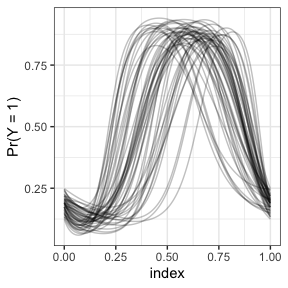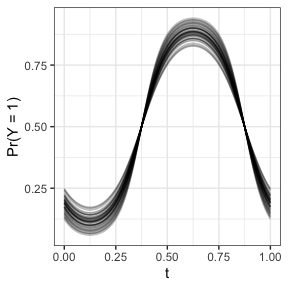Each curve has one main peak, but the location of that peak is shifted. When curves are registered the peaks are aligned.

### Simulate data for GFPCA

simulate_functional_data() simulates data with a population-level mean and two orthogonal principal components based on sine and cosine functions. The code below generates data with $$I = 100$$ subjects and $$D = 200$$ time points per subject using this function:

fpca_data = simulate_functional_data(I = 100, D = 200)

ls(fpca_data)
#>  "Y"     "alpha" "psi1"  "psi2"

head(fpca_dataY) #> id value index latent_mean #> 1 1 0 0.000000000 -0.33804471 #> 2 1 0 0.005025126 -0.27747105 #> 3 1 0 0.010050251 -0.21627513 #> 4 1 0 0.015075377 -0.15459419 #> 5 1 1 0.020100503 -0.09256655 #> 6 1 1 0.025125628 -0.03033132 The resulting object,fpca_data, is a list that contains the true population-level mean (alpha) and principal components (psi1 and psi2), and a dataframe (Y). The dataframe Y contains variables id, value, index and latent_mean. This data is plotted below.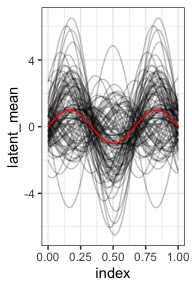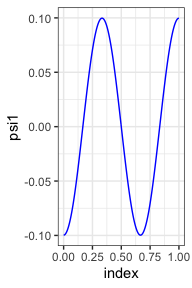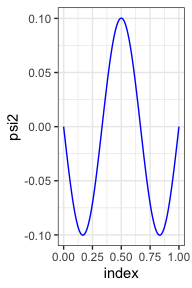The left panel of the figure above shows the latent means for each subject, along with the population-level mean, $$\alpha(t)$$, in red. The middle and right panels show the first and second principal components, $$\psi_1(t)$$ and $$\psi_2(t)$$, respectively. Using the $$logit^{-1}(\cdot)$$ function we can convert the subject-specific means to probabilities; these probabilities are used to generate the binary values. Binary values and latent probability curve for one subject in the dataset is shown below.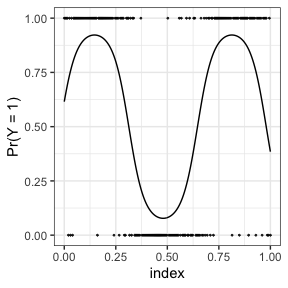We can alter the score variance for the principal components using the arguments lambda1 and lambda2. The default setting is for all subjects to have the same number of time points. However, by specifying vary_D = TRUE, we can generate data with uneven grid lengths for each subject. ## Registration using register_fpca() register_fpca() is the main function for the registr package. Use the family argument to this function to specify what type of exponential family data you would like to align. The package supports family = "binomial" for registering binary data and family = "gaussian" for registering continuous data. ### register_fpca(family = "binomial") for binary data To register binary data use the following code:  registr_bin = register_fpca(Y = registration_data, family = "binomial", Kt = 8, Kh = 3, npc = 1) The argument Y specificities the input dataset; this code uses the simulated registration_data. Kt and Kh specify number of B-spline basis functions for the subject-specific means and warping functions, respectively, and npc indicates the number of functional principal components to use.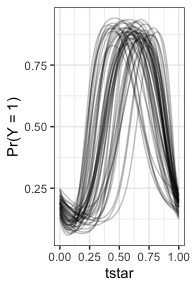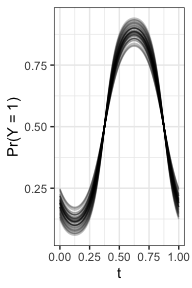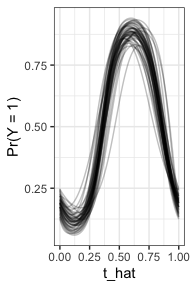ing the binary data are plotted above. At left probabilities on unregistered domain $$t^*$$, center are probabilities on true registered domain $$t$$, and at right are probabilities on estimated registered domain $$\widehat{t}$$. After registration the underlying probabilities are aligned – though it is important to note that the algorithm registers based on the underlying binary observations, not the true probabilities.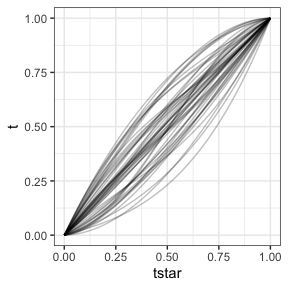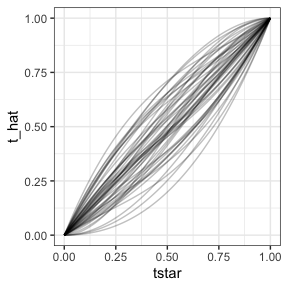The true an estimated warping functions are plotted above. ### register_fpca(family = "gaussian") for continuous data To register continuous data use the following code: Yvalue = Y$latent_mean registr_gauss = register_fpca(Y = registration_data, family = "gaussian", Kt = 10) ## bfpca() function The registr package includes a novel variational EM algorithm for binary functional principals component analysis (bfpca), derived from methods for binary probabilistic PCA (Tipping 1999). This bfpca() function works for data that is sparse and irregular (subjects do not have to be observed on the same grid and do not have to have the same number of grid points), as well as dense, regularly observed data. The following code runs bfpca on the fpca_data dataset. bfpca_object = bfpca(fpca_data$Y, npc = 2, Kt = 8, print.iter = TRUE)

The argument print.iter = TRUE prints the error after each iteration. The true and estimated population-level mean and FPCs are plotted below.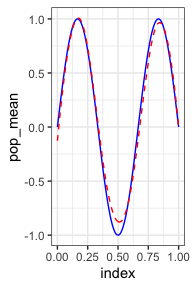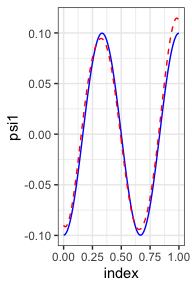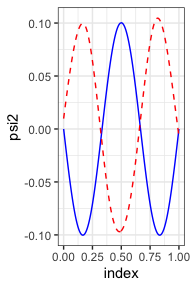The algorithm runs quickly and does a good job recovering the true FPCs. Note that while the truth and estimation are not perfectly aligned, this is to be expected – the data used to estimate these functions are binary observations that are generated for the truth with some variability, so results are not expected to perfectly align. One would expect results to get better with increasing number of time points per subject.

## registr() function

The registration step of register_fpca() calls the registr function. Though registration is intended to be performed through the register_fpca() function registr() can work as a standalone function. registr() uses constrained maximization of an exponential family likelihood function to estimate functions that align curves.

The default option gradient = TRUE implements an analytic gradient for this optimization problem. Selecting gradient = FALSE implements a numeric gradient which is less computationally efficient. This is illustrated in the code below.

data_test_gradient = simulate_unregistered_curves(I = 50, D = 100)

start_time = Sys.time()
end_time = Sys.time()

analytic_gradient = as.numeric(round((end_time - start_time), 2))

start_time = Sys.time()
numeric_gradient = as.numeric(round((end_time - start_time), 2))
On a dataset with just 50 subjects and 100 time points per subject, the registr() function runs in 1.56 seconds with an analytic gradient and 1.74 seconds with a numeric gradient. Since the register_fpca() algorithm is iterative and calls the registr() function several times, using an analytic derivative drastically increases the computational efficiency, especially if number of subjects in the dataset is large.
• ?register_fpca
• ?registr
• ?bfpca